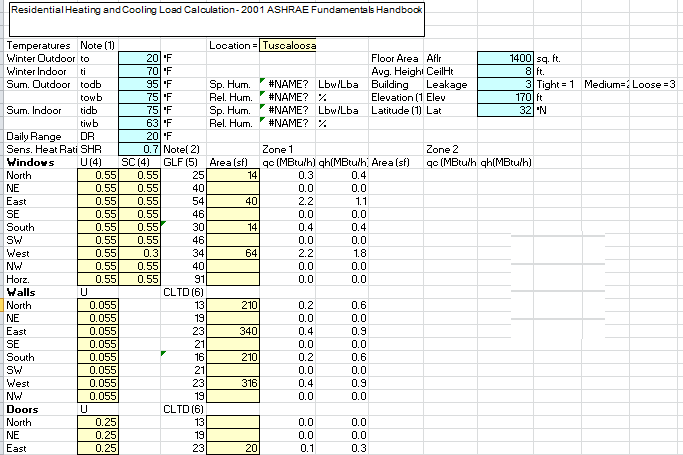ASHRAE Heat Load Calculation Excel Sheet

# ASHRAE Heat Load Calculation Excel Sheet

ASHRAE Heat Load Calculation Excel Sheet :This heating system sizing calculator is based on the ASHRAE Standards. this calculator will calculate Heating loads for air condioning systems for residential places.ASHRAE Heat Load Calculation

## ASHRAE Heat Load Calculation Excel Sheet

### Cooling Load Calculation For HVAC :

This Spreadsheet Calculates the following :
2. Duct quantities
3. ASHRAE Table- 5 Shading Coefficients and U - Factors for Residential Windows.

This heating system sizing calculator is based on the ASHRAE standards . this calculator will calculate Heating loads for air condioning systems for residential places.

### Important notes :

• Weather and climatic information available Table  1A & 1B . CHP. 27.201 ASHRAE Fundamental Handbook .
• SHR = Seneible Load / Total Load Use : 0.65 for Tropic, 0.70 Humid, .075 Avg., 0.80 Dry, 0.80 Arid
• Values Curve fit from Tables 7 & 8, chp. 28,2001 ASHRAE Fundamental Handbook.
• See Table 5, Chp. 28, 2001 ASHRAE Fundamental Handbook ( reproduced ona another Worksheet ).
• Values curve fit from Table1 , chp . 28,2001 ASHRAE fundamental Handbook.
• See Table 16 , chp.28, 2001 ASHRAE Fundamental Handbook.